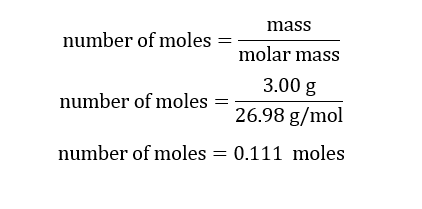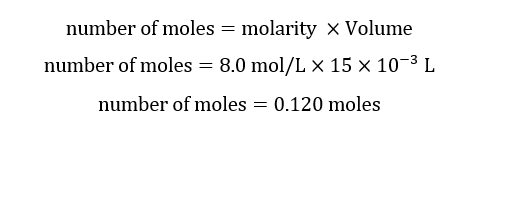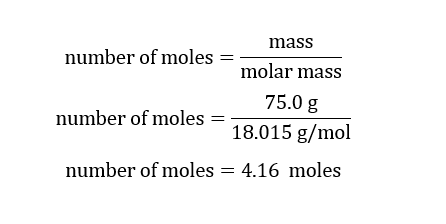# 2 Al (s) + 2 KOH (aq) + 6 HOH (l) ---> 2 KAl(OH)4 (aq) + 3 H2 (g)Under experimental procedures #2 and #3, the experiment calls for reacting aluminum with water and potassium hydroxide. See above for the equation. A student using quantities:3.00 grams Al15.0 mL of 8.00 M KOH75.0 mL distilled HOHWhich of the reactants is the limiting reactant? (Mathematically document this.) Show your work and set up.

Question
115 views

2 Al (s) + 2 KOH (aq) + 6 HOH (l) ---> 2 KAl(OH)4 (aq) + 3 H2 (g)

Under experimental procedures #2 and #3, the experiment calls for reacting aluminum with water and potassium hydroxide. See above for the equation. A student using quantities:

3.00 grams Al

15.0 mL of 8.00 M KOH

75.0 mL distilled HOH

Which of the reactants is the limiting reactant? (Mathematically document this.) Show your work and set up.

check_circle

Step 1

Given:

3.00 grams Al

15.0 mL of 8.00 M KOH

75.0 mL distilled H2O

Chemical reaction is given by,

2 Al (s) + 2 KOH (aq) + 6 HOH (l) ---> 2 KAl(OH)4 (aq) + 3 H2 (g)

Step 2

Calculation for moles of Al:Calculation for moles of KOH:Calculation for moles of H2O:

Mass = density × volume

Mass = 1 g/mL × 75.0 mL

Mass of H2O = 75.0 g...

### Want to see the full answer?

See Solution

#### Want to see this answer and more?

Solutions are written by subject experts who are available 24/7. Questions are typically answered within 1 hour.*

See Solution
*Response times may vary by subject and question.
Tagged in